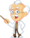Homework Explained - Math Practice 101Dear guest, you are not a registered member. As a guest, you only have read-only access to our books, tests and other practice materials.

As a registered member you can:

 Selected Response Question 1 A pizza parlor offers thin, thick, and traditional style pizza crusts. You can get pepperoni, beef, mushrooms, olives, or peppers for toppings. You order a one-topping pizza. How many outcomes are in the sample space? 3 5 8 15(show solution) Question 2 A bag contains 9 purple marbles, 2 blue marbles, and 4 pink marbles. The probability of randomly drawing a blue marble is $$\large\frac{2}{15}$$. What is the probability of not drawing a blue marble? $$\frac{2}{15}$$ $$\frac{4}{15}$$ $$\frac{11}{15}$$ $$\frac{13}{15}$$(show solution) Question 3 During the month of April, Dora kept track of the bugs she saw in her garden. She saw a ladybug on 23 days of the month. What is the experimental probability that she will see a ladybug on May 1? $$\frac{1}{23}$$ $$\frac{7}{30}$$ $$\frac{1}{2}$$ $$\frac{23}{30}$$(show solution) Question 4 Ryan flips a coin 8 times and gets tails all 8 times. What is the experimental probability that Ryan will get heads the next time he flips the coin? 1 $$\frac{1}{2}$$ $$\frac{1}{8}$$ 0(show solution) Question 5 A used guitar is on sale for $280. Derek offers the seller $$\large\frac{3}{4}$$ of the advertised price. How much does Derek offer for the guitar?$180 $210$240 \$270(show solution) Question 6 Jay tossed two coins several times and then recorded the results in the table belowWhat is the experimental probability that the coins will land on different sides on his next toss? $$\frac{1}{5}$$ $$\frac{2}{5}$$ $$\frac{3}{5}$$ $$\frac{4}{5}$$(show solution) Question 7 What is the probability of tossing two fair coins and having exactly one land tails side up? $$\frac{1}{8}$$ $$\frac{1}{4}$$ $$\frac{1}{3}$$ $$\frac{1}{2}$$(show solution) Question 8 Find the percent change from 60 to 96. 37.5% decrease 37.5% increase 60% decrease 60% increase(show solution) Question 9 A bag contains 6 white beads and 4 black beads. You pick out a bead, record its color, and put the bead back in the bag. You repeat this process 35 times. Which is the best prediction of how many times you would expect to remove a white bead from the bag? 6 10 18 21(show solution) Question 10 A set of cards includes 20 yellow cards, 16 green cards, and 24 blue cards. What is the probability that a blue card is chosen at random? 0.04 0.24 0.4 0.66(show solution)